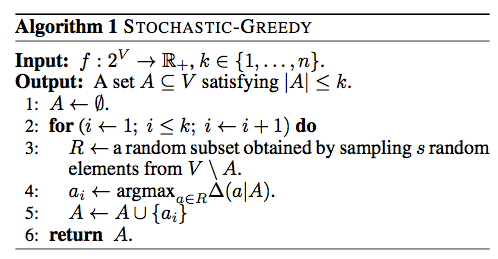# LIME - Local Interpretable Model-Agnostic Explanations

### Update

I've rewritten this blog post elsewhere, so you may want to read that version instead (I think it's much better than this one)

In this post, we'll talk about the method for explaining the predictions of any classifier described in this paper, and implemented in this open source package.

### Motivation: why do we want to understand predictions?

Machine learning is a buzzword these days. With computers beating professionals in games like Go, many people have started asking if machines would also make for better drivers, or even doctors.

Many of the state of the art machine learning models are functionally black boxes, as it is nearly impossible to get a feeling for its inner workings. This brings us to a question of trust: do I trust that a certain prediction from the model is correct? Or do I even trust that the model is making reasonable predictions in general? While the stakes are low in a Go game, they are much higher if a computer is replacing my doctor, or deciding if I am a suspect of terrorism (Person of Interest, anyone?). Perhaps more commonly, if a company is replacing some system with one based on machine learning, it has to trust that the machine learning model will behave reasonably well.

# Lazier than Lazy Greedy

We will be going step-by-step through this paper. Please refer back to this post for the definitions we need for submodularity and the greedy algorithm. The algorithm is as follows:# The greedy algorithm for monotone submodular maximization

First, some context:

### Submodular Functions

Submodular functions have the intuitive diminishing returns property. Formally, a submodular function $$f:2^V \rightarrow \mathbb{R}$$ assigns a subset $$A \subseteq V$$ a utility value $$f(A)$$ such that

$f(A \cup \{i\}) - f(A) \geq f(B \cup \{i\}) - f(B)$

for any $$A \subseteq B \subseteq V$$ and $$i \in V \setminus B$$. We call $$V$$ the ground set.

# Expectation Maximization

EM is a broad topic. I hope to just give a brief but understandable introduction to it here. This material is borrowed heavily from a class I took, taught by Mathias Drton.

$$\newcommand{\norm}{\left\| #1\right\|} \require{cancel}$$ Gradient descent is a very popular optimization method. In its most basic form, we have a function $$f : \mathbb{R}^n \rightarrow \mathbb{R}$$ that is convex and differentiable. We want to find:

$x^* = argmin_{x \in \mathbb{R}^n}f(x)$

First, some definitions:

### Lipschitz continuity

$$\newcommand{\norm}{\left\| #1\right\|}$$ A function $$f$$ is called L-Lipschitz over a set S with respect to a norm $$\norm{·}$$ if for all $$u,w \in S$$ we have:

# Why is the log likelihood of logistic regression concave?

Formal Definition: a function is concave if

$f((1-\alpha) a+ \alpha b) \geq (1-\alpha ) f(a)+\alpha f(b) \quad \forall a,b, \quad 0 \le \alpha \le 1$

# Is Logistic Regression a linear classifier?

A linear classifier is one where a hyperplane is formed by taking a linear combination of the features, such that one 'side' of the hyperplane predicts one class and the other 'side' predicts the other.

$$\newcommand{\norm}{\left\| #1\right\|}$$ A norm is a function (usually indicated by the vertical bars, such as $$\norm{\cdot}$$) such that for all $$w \in \mathbb{R}^n$$:
• $\norm{w} \geq 0$
•  For all $$w$$ and for all $$a \in \mathbb{R}$$, $$\norm{aw} = a \norm{w}$$. Note that$$ a $$denotes the absolute value of$$a$$
• $$\norm{w} = 0$$ if and only if $$w = 0$$. Note that 0 can be the zero vector of any length.
• For all $$u, w$$, $$\norm{u +w} \leq \norm{u} + \norm{w}$$. This is called the triangle inequality.